Name:    MCAS Conservation Laws - Energy

Multiple Choice
Identify the choice that best completes the statement or answers the question.

1.

The figure below shows a wagon that moves from point X to point Y. (MCAS 2006)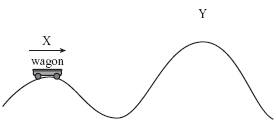Which of the following best describes the wagon’s change in energy as it coasts from point X to point Y?
 a. The wagon has the same kinetic energy at point Y and at point X. b. The wagon has more kinetic energy at point Y than at point X. c. The wagon has the same gravitational potential energy at point Y and at point X. d. The wagon has more gravitational potential energy at point Y than at point X.

2.

Which of the following describes the mechanical energy of a cart at rest at the top of a steep hill? (MCAS 2006)
 a. The cart has no mechanical energy. b. The cart’s mechanical energy is all kinetic. c. The cart’s mechanical energy is all potential. d. The cart’s mechanical energy is half potential and half kinetic.

3.

An astronaut drops a 1.0 kg object and a 5.0 kg object on the Moon. Both objects fall a total distance of 2.0 m vertically. Which of the following best describes the objects after they have fallen a distance of 1.0 m? (MCAS 2006)
 a. They have each lost kinetic energy. b. They have each gained the same amount of potential energy. c. They have each lost the same amount of potential energy. d. They have each gained one-half of their maximum kinetic energy.

4.

A cart at the top of a hill is released and rolls down the hill. Which of the following describes the energy of the cart just as it reaches the bottom of the hill? (MCAS 2007)
 a. The cart has no energy. b. The cart has maximum kinetic energy. c. The cart has maximum gravitational potential energy. d. The cart has equal gravitational potential and kinetic energy.

5.

A 9 kg model airplane flies horizontally at a constant speed. If the plane suddenly dives from its altitude of 50 m and levels off at 20 m, how much potential energy does it lose in the dive? (MCAS 2007)
 a. 450 J c. 2700 J b. 1800 J d. 9000 J

6.

Three different boxes are lifted to different heights. (MCAS 2008)
Box X weighs 115 N and is lifted to 15 m.
Box Y weighs 210 N and is lifted to 10 m.
Box Z weighs 305 N and is lifted to 5 m.
Which of the following statements best describes the boxes’ change in mechanical energy?
 a. Box X had the greatest change in mechanical energy. b. Box Z had the smallest change in mechanical energy. c. Boxes X and Y had the same change in mechanical energy. d. Boxes Y and Z had the same change in mechanical energy.

7.

Which of the following increases when a metal spring is stretched horizontally? (MCAS 2008)
 a. potential energy c. gravitational energy b. kinetic energy d. electrical energy

8.

The diagrams below show a man swinging a golf club.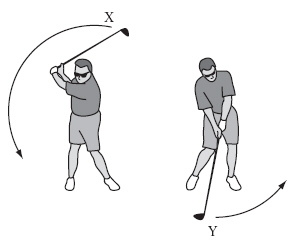The kinetic energy of the golf club at point Y is more than the potential energy of the club at point X. Which of the following statements best explains why this fact does not violate the law of conservation of energy? (MCAS 2009)
 a. Gravity is constant from point X to point Y. b. Air resistance is greater at point Y than at point X. c. Acceleration due to gravity is greater at point Y than at point X. d. Energy is added by the man to the golf club from point X to point Y.

9.

The figure below represents a pendulum’s motion with the lowest point of its swing labeled P.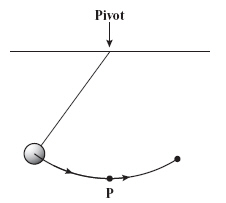What happens to most of the pendulum’s gravitational potential energy as it reaches the lowest point P? (MCAS 2009)
 a. It is transformed into inertia. c. It is transformed into thermal energy. b. It is transformed into kinetic energy. d. It is transformed into chemical energy.

10.

The diagram below shows the path of a student on a sled starting from rest at point W.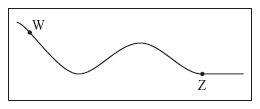The student slides down a frictionless, snow-covered hill past point Z, which is at ground level.
Which of the following statements best describes the energy of the student and sled from point W to point Z? (MCAS 2009)
 a. The total energy at point W is less than at point Z. b. The total energy at point W is greater than at point Z. c. The potential energy at point W becomes all kinetic energy at point Z. d. The kinetic energy at point W becomes all potential energy at point Z.

11.

A skateboarder travels from location 1 to location 4 as shown below.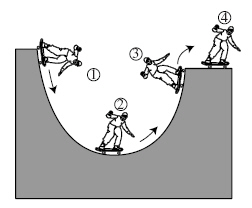At which location does the skateboarder have the most kinetic energy and the least potential energy? (MCAS 2004)
 a. 1 c. 3 b. 2 d. 4

12.

A student swings a bat horizontally, making contact with a ball thrown to her. The ball leaves the bat, and the bat continues moving through the rest of the swing. Which of the following statements describes the change in energy necessary to do work on the ball? (MCAS 2010)
 a. All the kinetic energy of the bat is converted to work. b. All the potential energy of the bat is converted to work. c. Some of the kinetic energy of the bat is converted to work. d. Some of the potential energy of the bat is converted to work.

13.

A ball is thrown straight upward. The ball’s initial speed is 30 m/s and its mass is 0.05 kg, resulting in an initial kinetic energy of 22.5 J. If the initial potential energy of the ball is 10 J and there is no frictional force, what would be the ball’s total energy while it is moving? (MCAS 2010)
 a. 0.0 J c. 22.5 J b. 10.0 J d. 32.5 J

14.

The diagram below represents a bicyclist at the top of a hill, with four points labeled W, X, Y, and Z.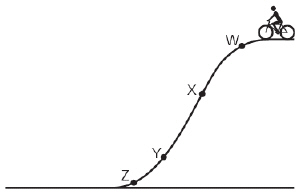Assume that the bicyclist does not apply the brakes as he rides down the hill. At which point will the bicyclist’s kinetic energy be closest to zero? (MCAS 2010)
 a. point W c. point Y b. point X d. point Z

15.

A pendulum swings back and forth several times, as shown in the diagram below. Assume friction is negligible.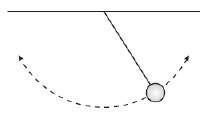Which of the following graphs best illustrates the potential energy (PE) and the kinetic energy (KE) of the pendulum over a period of several swings? (MCAS 2011)
 a.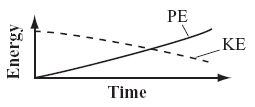b.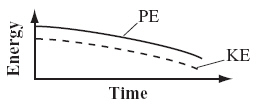c.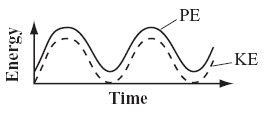d.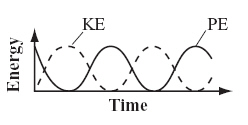16.

A 5 kg rock and a 10 kg rock are dropped from a height of 10 m. Which of the following statements describes what happens to the potential energy of the rocks? (MCAS 2011)
 a. Neither rock loses potential energy because no work is done on the rocks. b. Both rocks lose the same amount of potential energy because they fall from the same height. c. The 10 kg rock loses more potential energy than the 5 kg rock because the 10 kg rock falls faster. d. The 10 kg rock loses more potential energy than the 5 kg rock because the 10 kg rock has more mass.

17.

An inventor claims to have designed a perpetual motion machine, a device that creates its own power. Which of the following laws best explains why a perpetual motion machine cannot work? (MCAS 2012)
 a. law of conservation of energy c. Newton’s second law b. law of conservation of matter d. Newton’s third law

18.

A 1000 kg automobile is traveling at an initial speed of 20 m/s. It is brought to a complete stop in 5 s over a distance of 50 m. What is the work done in stopping the automobile? [Remember - work is equal to change in energy] (MCAS 2012)
 a. 10,000 J c. 50,000 J b. 40,000 J d. 200,000 J

19.

While playing a game at a fair, a person lifts a mallet above her head. She then lets the mallet fall toward a target, as shown below.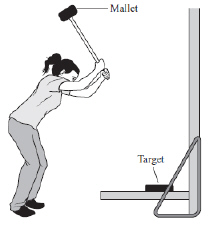Which of the following statements describes an energy change that takes place as the person lifts the mallet and then lets it fall toward the target? (MCAS 2012)
 a. Kinetic energy increases as the mallet reaches its highest point. b. Potential energy decreases as the mallet reaches its highest point. c. Kinetic energy converts to potential energy as the mallet falls toward the target. d. Potential energy converts to kinetic energy as the mallet falls toward the target.

20.

The potential energy of a 77 kg diver standing on a 20 m high diving tower is 15,400 J. Two-thirds of the way down during the dive into the pool, his potential energy is 5,100 J. Neglecting air resistance, what is the diver’s kinetic energy at this point? (MCAS 2013)
 a. 2,550 J c. 10,300 J b. 7,650 J d. 12,850 J

21.

Work is performed on an object by raising it 2 m above the floor. Which of the following types of energy must change in this situation? (MCAS 2013)
 a. chemical energy c. mechanical energy b. magnetic energy d. thermal energy

22.

Neglecting air resistance, which of the following statements describes the change in energy of a diver who falls downward toward a swimming pool? (MCAS 2014)
 a. Potential energy increases, kinetic energy increases, and total energy increases. b. Potential energy decreases, kinetic energy decreases, and total energy decreases. c. Potential energy decreases, kinetic energy increases, and total energy remains the same. d. Potential energy increases, kinetic energy decreases, and total energy remains the same.

23.

A 4000 kg truck is moving north at 8 m/s, and a 1200 kg car is moving north at 30 m/s. Which of the following statements best compares the truck and the car? (MCAS 2014)
 a. The truck has less inertia than the car. b. The truck has less momentum than the car. c. The truck has more mass but less weight than the car. d. The truck has more kinetic energy but less potential energy than the car.

24.

The diagram below shows a section of a roller coaster track. Five points on the track are labeled.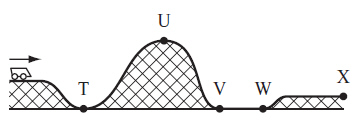Between which two points will the roller coaster’s gravitational potential energy be converted into kinetic energy? (MCAS 2014)
 a. T and U c. V and W b. U and V d. W and X

Essay

25.

In the diagram below, the falling water turns the waterwheel. The turning waterwheel generates electricity. (MCAS 2008)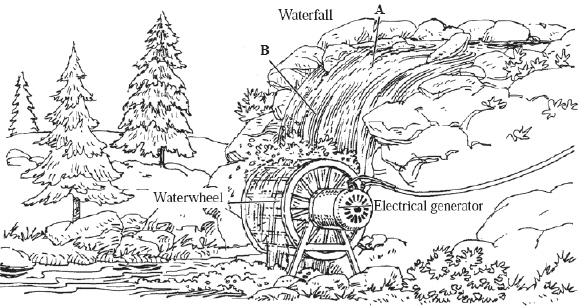The water moves slowly at point A and then falls rapidly past point B.

a. Describe the changes in kinetic and gravitational potential energy of the water as it travels from point A to point B.

b. Explain why not all of the energy of the moving water available at point A is captured by the waterwheel to generate electricity.

c. Describe two ways the system can be changed so that more energy from the falling water is converted into electrical energy.

26.

The figure below shows a tennis ball bouncing from point 1 to point 5. (MCAS 2005)The tennis ball bounces up from point 1 as shown in the as point 2. The ball then bounces a few times. Neglect any horizontal motion.

a. Describe the kinetic and gravitational potential energy changes of the ball that occur between points 1 and 2.

b. Describe the kinetic and gravitational potential energy changes of the ball that occur between points 2 and 3.

c. Compare the kinetic energy of the ball at points 3 and 4.

d. The tennis ball has less energy at point 5 than it had at point 3. Explain what happened to the energy the ball had at point 3.

27.

A pendulum is made with a bowling ball as the bob and a wire attached to the ceiling, as shown in the illustration below. The person in the illustration pulls the bowling ball back until it touches his nose, then releases the bowling ball. Assume that he does not move. (MCAS 2010)a. Describe the changes in the potential energy and the kinetic energy of the bowling ball as it swings back and forth.
b. Explain whether the bowling ball will hit the person’s nose. Include a discussion of energy changes in your explanation.
c. Explain what will happen if the person gives the bowling ball a small push as it is released. Include a discussion of energy changes in your explanation.

28.

Students in a physics class are studying the energy changes that objects experience as they fall. The students plan to drop a metal sphere with a mass of 0.05 kg from a height of 20.0 m onto a bed of sand. They predicted the results shown in the table below. (MCAS 2011)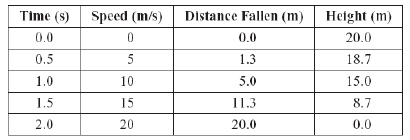a. Using the predicted values in the table, calculate the kinetic energy of the sphere just before it contacts the sand at 2.0 s. Show your calculations and include units in your answer.

b. Using the predicted values in the table, calculate the gravitational potential energy at 0.0 s. Show your calculations and include units in your answer.

c. Describe what happens to the gravitational potential energy and the kinetic energy as the sphere falls.

After the sphere is dropped, the students find that the results of the experiment are slightly different than they predicted.

d. Assuming the students performed their calculations correctly, explain one possible reason for the difference they observed.

29.

A 70 kg person is swinging on a swing set, as shown in the diagram below. Positions X and Z represent the highest points of the person’s motion, and position Y represents the lowest point of the person’s motion. (MCAS 2013)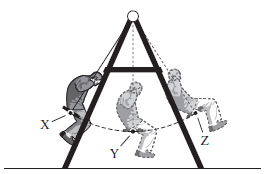a. At which position does the person have maximum kinetic energy? Explain your answer.
b. Neglecting friction, describe the energy conversion as the person travels from position X to position Y.
c. The person is 1.0 m above the ground at position Y and 1.5 m above the ground atposition Z. Neglecting friction, calculate the change in gravitational potential energy as the person swings from position Y to position Z. Show your calculations and include units in your answer.
d. Neglecting friction, calculate the speed of the person at position Y. Show your calculations and include units in your answer.